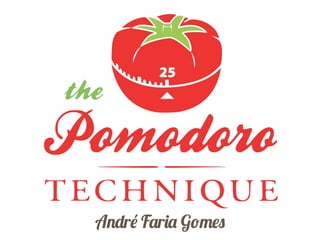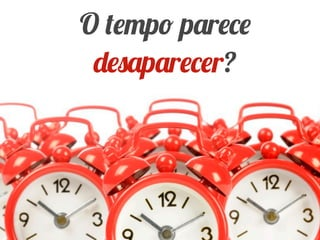Successfully reported this slideshow.

# Pomodoro

10

Share×
1 of 75
1 of 75

# Pomodoro

10

Share

A nice introduction to the pomodoro technique by André Faria.

A nice introduction to the pomodoro technique by André Faria.

## More Related Content

### Related Books

Free with a 14 day trial from Scribd

See all

### Related Audiobooks

Free with a 14 day trial from Scribd

See all

### Pomodoro

1. 1. A r F r G
2. 2. O v v ê ã f r r ?
3. 3. O p p r p r r?
4. 4. V ê p p pr p rf q f v v r f rf ? T !
5. 5. S Pr b A b r ...
6. 6. P r r XP Sr v r . Sã r çõ 25 1p . H r K br
7. 7. C p D v Pr r v çã v Q , pr b v ... ã r r v q
8. 8. Pr r çã q rf ã
9. 9. çã P r r M p r r ç r r r rf ã . F Pr r çã A f rç f r q v ê ã q r. S pr pr pr ã p r f r p rf . M r rr r br r .
10. 10. E r
11. 11. z p :S r q r rp r 10 ?
12. 12. 1. E rf rv . p p r 25 . 2. D p r r . 3. Tr b p br v 5 . 4. A 4P r, p . 5. A
13. 13. OT p C v ê r ?
14. 14. N çã T p M
15. 15. N çã T p S v
16. 16. Pr P r • U f r fr vr p , ã b , ã v v r ê . • U r f q r p , ê , rf , f pr . • Ap r f rr ã r v r z p p r f v r pr q v ê r r v q v ê q r r. M f p r r r p rf ã .
17. 17. O 5E A I r çã D r I : P r T q I r (Pr Pr )
18. 18. P N I D ,p r D r v ,v ê p p r b rv v .
19. 19. r M
20. 20. R r r p r p r N . rv çõ rq v r
21. 21. Pr Ap r r , r f r r br f r çã , p r p vr rr pçõ p rp r .
22. 22. V r N ,p r pr r f r çã f r q f r p r r r .
23. 23. P D C A
24. 24. M r T r B
25. 25. OP r I v v
26. 26. P
27. 27. N Pr
28. 28. O Pr r P r
29. 29. OS
30. 30. A Q r
31. 31. C p A v
32. 32. Q br p •S v ê r r rf pr r rq rv ã ã v p , ã p r v . • Apr v br p p r vr r .
33. 33. r r Ov E r pr r p q r r pr ê
34. 34. Gr v
35. 35. A A • Oq f v êp r r rf r - ? • Oq v êf p r ã ç r rf ? •E q p v v ê r v p p r r r q ?
36. 36. I rr pçõ
37. 37. E -
38. 38. I r •N rr p ! • L v rp r p r p r r •F r çã q p r r • r r r - •p r r rf • Sã r çõ f r pr r r r b ã ...
39. 39. V I r •Tr pr b v v •S pr q p rr pçã v r b ç , rq p r f . •E rv rr pçã T D S Nã p Ur . •E rv q v U ã p . •Tr rf , r b r r q p r q ...
40. 40. C Pr •E v r b r br q q r p r C r ... p pr b q p rf p .( p p)
41. 41. P z
42. 42. N I rr pçõ V êp v rp r q v , f r p , rf r .
43. 43. I rr p P r •S v ê rp r r p r p r f r r , v r p r . Nã p p r r p . • L br - r r : • OP r I v v !
44. 44. A • P rq p v êp f r v r ? •Q ã r v p pr ? •V ê pr r ?N Q ?P rq ?
45. 45. r •Pr çõ • L A •P
46. 46. I vrã Dp ê •A fr ç r r r q , v ê pr r r p .P r r f ç q r , vr p ê ,p r p r v ê. • U p r • D v - , , ... r p r • D p q v êf r r . • E r ê r ã r r ,q p p r r 25 p r .P r q pr f p f r ç ,p r v êf .
47. 47. I rr pçõ •A r r p 10 15 rr pçõ r p rp r . •S v ê pr r r p , rã pr r p b v v r rã r p . •M p pr br q r p 3p r p r p r r b r , r r r õ , f , - , .
48. 48. V b •A rr pçã r r ç . • S f rp r , rv v v Nã P Ur , pr rv q U pr . •C P r A p rq r ç p r .
49. 49. L r •If r r: E •N r: P f r v ê 15 ? •A r: E r v v p p p r r p r . •R r :R r v ê pr ã v pr r ç p .
50. 50. V b
51. 51. A A • C v ê rr p r b ? • C v ê q rr v ? • C q r q f r v ? • V ê pr r r r q pr ?
52. 52. ê pr v v V f r p q , p r ! ã
53. 53. V ê p r p pr r p r 15 5, br - q fr ã p v .
54. 54. C ... O C b q br q N ,v ê P r r q ã v r
55. 55. E
56. 56. E v • N vr q q p r q v ê f r v pr r.
57. 57. E r A r M p
58. 58. T b r-P ã -C r • T b r: O r p r p r • P ã :O r v b • C r :Q p ã v r ,q r f .A b çõ v r v .
59. 59. E v •N 1/2 P r •C v q pr 5 p r , q br - r . •V êp b r v :
60. 60. P D r A r q v ê b q p r p r ,f ç p r .
61. 61. E R R- q v ç
62. 62. A
63. 63. L p D v q v ê v f r p q r q ã r .
64. 64. R C •C rr v q r b q rã . •U r q r çã p .O ã pr r r . •V ê p v r ? •F r r rq vr f p r p r r r r b !
65. 65. A A •C q fr qüê v êf p r b ? •C q fr qüê v êr br q f ?
66. 66. B “É p r b r A r v , v .” I : P r T q I r (Pr Pr )
67. 67. D r çã Gr r rf r r rr pçõ v r v r rf q f rç v .
68. 68. “F r v ê p rq b f r b r p ”
69. 69. F b Pr p r r v pr b r pr r , r b r p r rf p r r ã pr f b r p . OP r pr q ç 25 .
70. 70. A p T p P r T p Br S C r çã ( ) r b ! P A p ,
71. 71. Tr b Eq p P r pr vr çã v ê p p rp r ã rp r br rr pçõ .
72. 72. õ b R Pr r v r ã f ç p r p r pr r . F ç p ...
73. 73. Pr r çã P r P D r • v p r r r f • C pr • A b r P rP • P P
74. 74. Rfr • L vr ê • G Ev r D • N w H bb • P r T q • P r T q I r (Pr Pr ) • L • P r I • p://www. b. /w ?v= H-z5 V Uz • Or v • p://www.v r. / p r/ r v/v /4/
75. 75. I •F •S I • p://www. r. /p • p